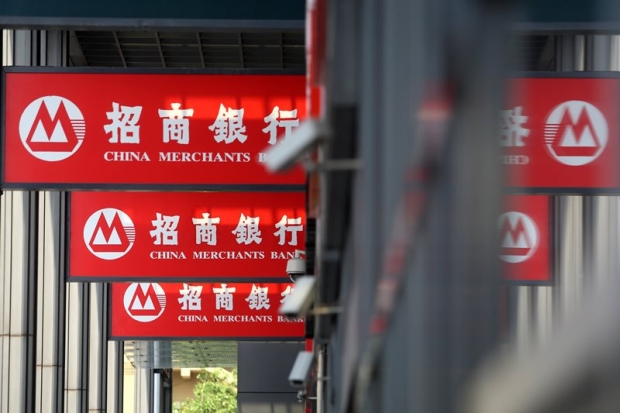# China Merchants Bank's medium-term earnings increased by 14%, non-performing loans declined – Newsletter website hkej.comChina Merchants Bank (03968) announced that it had an interim profit of 44.756 billion yuan (RMB ‧ the same) as of the end of June, an increase of 14% on an annual basis and a profit per share of 1.77 yuan, no dividends.

Over the period, net interest income amounted to 77.012 billion yuan, an increase of 8.63% on an annual basis; net commission income amounted to 37.69 billion yuan, an increase of 7.82% on an annual basis. Net interest margin and net interest margin were 2.42% and 2.54% respectively, both 0.11 percentage points year-on-year.

At the end of June total non-performing loans totaled 55.322 billion yuan, a decrease of 2.011 billion yuan at the end of last year, the non-performing loan ratio 1.43%, a decrease of 0.18 percentage points at the end of last year, while the funding ratio of the non-performing loans amounted to 316.08%, an increase of 53,97 percentage points compared to the end of last year.

The core tier 1 solvency ratio of China Merchants Bank was 11.61%, a decrease of 0.45 percentage points compared to the end of last year: the tier 1 solvency ratio was 12.51%, a decrease of 0.51 percentage points compared to the end of last year.

# China Merchants Bank's medium-term earnings increased by 14%, non-performing loans declined – Newsletter website hkej.comChina Merchants Bank (03968) announced that it had an interim profit of 44.756 billion yuan (RMB ‧ the same) as of the end of June, an increase of 14% on an annual basis and a profit per share of 1.77 yuan, no dividends.

Over the period, net interest income amounted to 77.012 billion yuan, an increase of 8.63% on an annual basis; net commission income amounted to 37.69 billion yuan, an increase of 7.82% on an annual basis. Net interest margin and net interest margin were 2.42% and 2.54% respectively, both 0.11 percentage points year-on-year.

At the end of June total non-performing loans totaled 55.322 billion yuan, a decrease of 2.011 billion yuan at the end of last year, the non-performing loan ratio 1.43%, a decrease of 0.18 percentage points at the end of last year, while the funding ratio of the non-performing loans amounted to 316.08%, an increase of 53,97 percentage points compared to the end of last year.

The core tier 1 solvency ratio of China Merchants Bank was 11.61%, a decrease of 0.45 percentage points compared to the end of last year: the tier 1 solvency ratio was 12.51%, a decrease of 0.51 percentage points compared to the end of last year.